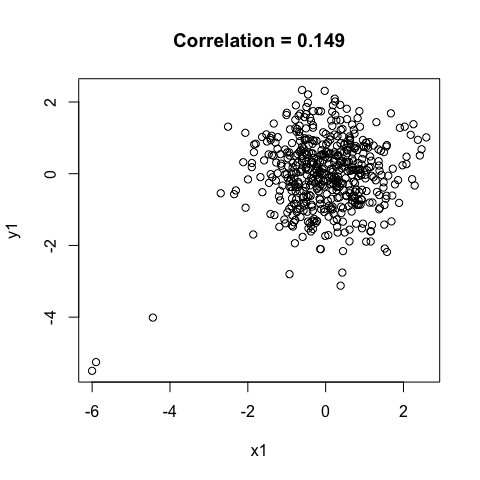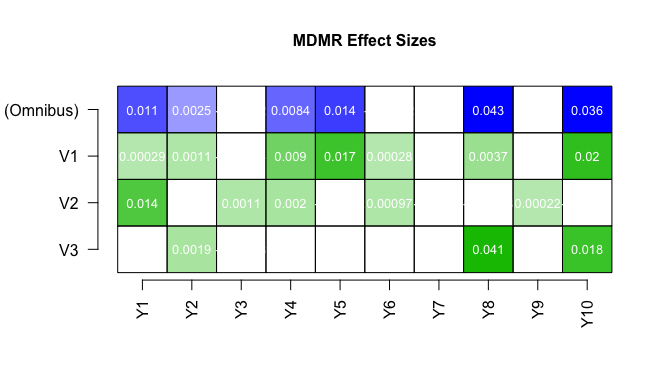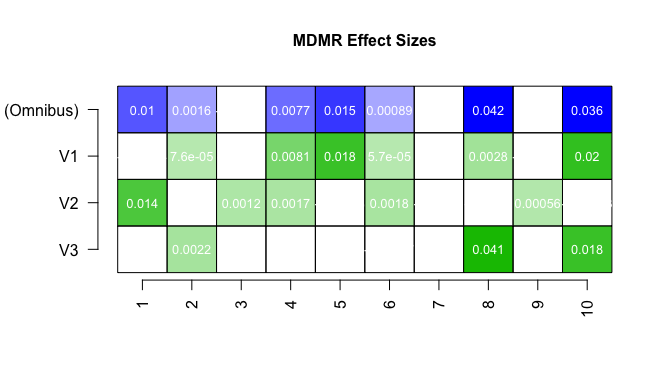Using the MDMR Package

2018-02-13

Many research questions involve the collection of multivariate outcome data. A common goal in these scenarios is the identification of predictors $$\left(\mathbf{X}_{n \times p}\right)$$ that are associated with the multivariate outcome $$\left(\mathbf{Y}_{n \times q}\right)$$. Methods such as multivariate multiple regression (MMR) and multivariate analysis of variance (MANOVA) are typical approaches to accomplishing this goal.

Multivariate distance matrix regression (MDMR; Anderson, 2001; McArdle & Anderson, 2001) is another method for identifying predictors that are associated with a multivariate outcome. MDMR is a two-step procedure that first computes the (dis)similarity between each pair of subjects’ multivariate respnose profiles, that is, the distance between each pair subjects’ scores along all variables comprising $$\mathbf{Y}$$. Importantly, any metric of (dis)similarity can be used to quantify the distance between response profiles (e.g. Euclidean, Manhattan), making MDMR a flexible and robust alternative to MMR and MANOVA.

These pair-wise distances are arranged into a symmetric $$n \times n$$ distance matrix $$\left(\mathbf{D}\right)$$, and MDMR tests the association between $$\mathbf{X}$$ and $$\mathbf{D}$$ by decomposing the sums of squares of the distance matrix into a portion attributable to regression onto $$\mathbf{X}$$ and a portion due to residual.

The MDMR package implements multivariate distance matrix regression in R using new developments to the method provided by McArtor et al. (second revision under review). In particular, MDMR provides analytical p-values for the MDMR test statistics, whereas previous implementations relied on permutation-based p-values because the null distribution of the test statistic was unknown. Furthermore, MDMR implements a new measure of effect size that uses a pseudo-jackknife procedure to quantify the association between each item comprising $$\mathbf{X}$$ and each item in $$\mathbf{Y}$$ that contributed to the construction of $$\mathbf{D}$$.

The remainder of this vignette is designed to illustrate the use of the MDMR package. For further technical details of the methods being implemented, please refer to McArtor et al.

1. Conducting MDMR

To illustrate this package, we include the toy dataset mdmrdata, which is comprised of ten continuous outcome variables $$\mathbf{Y}$$ and three continuous predictors $$\mathbf{X}$$ measured on 500 subjects. All variables are standardized to mean zero and unit variance. We will use robust Manhattan distances to construct the distance matrix outcome $$\mathbf{D}$$ by using the dist() function in R.

library(MDMR)
data(mdmrdata)
D <- dist(Y.mdmr, method = "manhattan")

The function mdmr() can be used in two ways to regress $$\mathbf{D}$$ onto $$\mathbf{X}$$.

1.1 Option A: Passing the Distance Matrix

The simplest approach is to pass the matrix of predictors and distance matrix outcome (either as a dist object or a matrix) to the function mdmr,

mdmr.res <- mdmr(X = X.mdmr, D = D)
summary(mdmr.res)
##           Statistic Numer.DF Pseudo.R2 Analytic.p.value
## (Omnibus)    0.1514        3    0.1315          < 1e-20 ***
## X1           0.0629        1    0.0546          < 1e-13 ***
## X2           0.0249        1    0.0217       3.2038e-06 ***
## X3           0.0685        1    0.0594          < 1e-13 ***
## ---
## Signif. codes:  0 "***" 0.001 "**" 0.01 "*" 0.05 "." 0.1 " " 1

The output is a data frame comprised of three columns and $$p+1$$, in this case four, rows. The first column provides the test statistic for the omnibus effect of all predictors on the distance matrix (first row) and the conditional effects of each predictor (remaining rows). The second column lists the omnibus and conditional pseudo-$$R^2$$ statistics, which are defined as the proportion of total sums of squares of $$\mathbf{D}$$ attributable to each corresponding effect. The third column lists the analytical p-value corresponding to that predictor.

1.2 Option B: Passing Gower’s Trannsformed Distance Matrix

In lieu of passing $$\mathbf{D}$$ to mdmr(), the user can supply the Gower-transformed distance matrix $$\mathbf{G}$$ (Gower, 1966; McArdle & Anderson, 2001; McArtor et al., second revision under review) that is used directly in the MDMR test statistic, as well as the eigenvalues of $$\mathbf{G}$$. This approach is advisable when mdmr() will be called multiple times on the same distance matrix using different sets of predictors. Analytical p-values require the computation of the eigenvalues of $$\mathbf{G}$$, and the time required to find the eigenvalues of an $$n \times n$$ matrix is proportional to $$n^3$$. This can become computationally expensive with large sample sizes, so it is best practice to compute them only once rather than each time mdmr() is called, as is done in Section 1.1.

Two options for implementing this procedure are illustrated below,

# --- Directly compute the eigenvalues
G <- gower(D)
lambda <- eigen(G, only.values = T)$values mdmr.res2 <- mdmr(X = X.mdmr, G = G, lambda = lambda) summary(mdmr.res2) ## Statistic Numer.DF Pseudo.R2 Analytic.p.value ## (Omnibus) 0.1514 3 0.1315 < 1e-20 *** ## X1 0.0629 1 0.0546 < 1e-13 *** ## X2 0.0249 1 0.0217 3.2038e-06 *** ## X3 0.0685 1 0.0594 < 1e-13 *** ## --- ## Signif. codes: 0 "***" 0.001 "**" 0.01 "*" 0.05 "." 0.1 " " 1 # --- Output the eigenvalues of G using the first call to mdmr() and pass them to # --- subsequent calls # Generate a hypothetical additional predictor we want to test first set.seed(102) x1 <- rnorm(500) mdmr.tmp <- mdmr(X = x1, D = D, return.lambda = T) # Pass the eigenvalues output by mdmr(return.lambda = t) to the next call of mdmr() lambda <- mdmr.tmp$lambda
mdmr.res3 <- mdmr(X = X.mdmr, G = G, lambda = lambda)
summary(mdmr.res3)
##           Statistic Numer.DF Pseudo.R2 Analytic.p.value
## (Omnibus)    0.1514        3    0.1315          < 1e-20 ***
## X1           0.0629        1    0.0546          < 1e-13 ***
## X2           0.0249        1    0.0217       3.2038e-06 ***
## X3           0.0685        1    0.0594          < 1e-13 ***
## ---
## Signif. codes:  0 "***" 0.001 "**" 0.01 "*" 0.05 "." 0.1 " " 1

All three approaches yield identical results. While the method in Section 1.1 is more convenient, it may add avoidable computation time if $$n$$ is large, and differentiating between the methods illustrated in Section 1.2 is simply a question of user preference.

2. Effect Sizes

In light of a statistically significant association between a predictor variable and $$\mathbf{D}$$, it will often be a substantive interest to identify which items in the outcome space are primarily driving this association. That is, it is often important to identify which variables comprising $$\mathbf{Y}$$ are associated with $$\mathbf{X}$$ in a manner that leads to a significant MDMR test statistic.

Post-hoc analyses such as correlations should not be used to answer this question under most circumstances because they do not take into consideration how the distance matrix was constructed. For example, one outcome item might have a high correlation with a predictor due to a few extreme outlying observations, but a robust distance metric may have been used that would not be driven by these outlying observations. This is the case here with $$\mathbf{x}_1$$ and $$\mathbf{y}_1$$:

plot(X.mdmr[,1], Y.mdmr[,1],
main = paste0("Correlation = ", round(cor(X.mdmr[,1], Y.mdmr[,1]), 3)),
xlab = "x1", ylab = "y1")cor.test(X.mdmr[,1], Y.mdmr[,1])
##
##  Pearson's product-moment correlation
##
## data:  X.mdmr[, 1] and Y.mdmr[, 1]
## t = 3.3605, df = 498, p-value = 0.0008379
## alternative hypothesis: true correlation is not equal to 0
## 95 percent confidence interval:
##  0.06202605 0.23354771
## sample estimates:
##       cor
## 0.1489067

A post-hoc test of this correlation might lead a researcher to conclude that the relationship between $$\mathbf{x}_1$$ and $$\mathbf{y}_1$$ is a substantial driver of the significant association between $$\mathbf{x}_1$$ and $$\mathbf{D}$$, but this is not the case, as will be shown below. The $$\mathbf{D}$$ matrix was constructed with Manhattan distances, which are robust against outliers. It is important to use an effect size measure that takes into consideration the structure of $$\mathbf{D}$$ if one is to infer which univariate effects are driving associations between $$\mathbf{X}$$ and $$\mathbf{D}$$.

The MDMR package implements the $$\delta$$ statistic proposed by McArtor et al. (second revision under review), which measures the effect size on each variable comprising $$\mathbf{Y}$$ through a pseudo-jackknife procedure that respects the distance metric used to construct $$\mathbf{D}$$. It measures the effect on each variable comprising $$\mathbf{Y}$$ by dissociating one outcome variable at a time from the predictors, constructing a new distance matrix with the remaining outcome variables as well as the dissociated outcome of interest, and regressing this new distance matrix onto $$\mathbf{X}$$. The extent to which the pseudo-$$R^2$$ from this regression decreases relative to the estimate of pseudo-$$R^2$$ from regressing $$\mathbf{D}$$ onto $$\mathbf{X}$$ measures the effect on the dissociated outcome variable. For more details, see McArtor et al.

Like the MDMR test statistics, $$\delta$$ statistics exist for the omnibus effects and conditional effects of each predictor comprising $$\mathbf{X}$$. The omnibus effect size on each $$\mathbf{y}_k$$ $$(k = 1, ..., q)$$ measures the effect size on each outcome variable, and is analogous to $$R^2$$ in multiple regression. The conditional effects of each predictor on each outcome variable measure the univariate effect of each predictor on each outcome, conditional on the rest of the predictors, and are therefore analogous to squared multiple regression coefficients. Importantly, $$\delta$$ is a relative measure of effect size, so estimates cannot be compared across studies. Instead, larger estimates of $$\delta$$ indicate larger effects and smaller estimates of $$\delta$$ indicate smaller effects (with negative estimates interpreted as virtually no effect). See McArtor et al. for more details.

There are two methods to use the MDMR package to compute $$\delta$$. The first should be used if the distance metric used to construct $$\mathbf{D}$$ is implemented in the dist() function in R. The second is more flexible but more tedious, and is intended for use with distance matrices that cannot be computed with a call to dist().

2.1 Option A: Passing Y

The first option to compute $$\delta$$ statistics it to pass the matrix of predictors, matrix of outcome data, and distance metric to the function delta(). The additional argument niter determines how many times the pseudo-jackknife procedure is conducted and averaged, such that larger numbers yield more stable estimates but require more computation time. See McArtor et al. for more details on niter.

Calling delta() returns a data frame of the $$\delta$$ statistics whose rows correspond to omnibus and conditional effects on the columns, which each correspond to a variable comprising the multivariate outcome. If the argument plot.it = T, as is default, a heatmap will also be displayed to easily visualize the largest effects.

par(mar = c(5, 5, 4, 2) + 0.1)
delta(X = X.mdmr, Y = Y.mdmr, dtype = "manhattan", plot.res = T,
niter = 1, seed = 12345)##                Y1.jack       Y2.jack       Y3.jack      Y4.jack
## (Omnibus)  0.011394518  2.493347e-03 -0.0002236513  0.008411022
## V1         0.000288711  1.087839e-03 -0.0002394429  0.009017106
## V2         0.013812441 -1.080107e-05  0.0010913754  0.001985751
## V3        -0.002127925  1.854581e-03 -0.0008780740 -0.002021005
##                 Y5.jack       Y6.jack       Y7.jack       Y8.jack
## (Omnibus)  0.0138529967 -0.0003211333 -0.0037978729  0.0425599922
## V1         0.0171844610  0.0002837745 -0.0016215904  0.0036666514
## V2        -0.0001189534  0.0009746623 -0.0004547291 -0.0003835555
## V3        -0.0027693726 -0.0012981087 -0.0018242033  0.0406426913
##                 Y9.jack      Y10.jack
## (Omnibus) -0.0028530015  0.0361314288
## V1        -0.0010690841  0.0199830137
## V2         0.0002199023 -0.0003141193
## V3        -0.0020442272  0.0176801735

There are several things to note here. First, it is clear that each predictor is affecting only a small subset of the predictors, and that not all outcome variables are affected (e.g. $$\mathbf{y}_3$$). Second, we see that the relationship between $$\mathbf{x}_3$$ and $$\mathbf{y}_8$$ is the strongest of all univariate effects, and only a few other univariate effects are notable (those heavily shaded in the figure). Third, the omnibus effects tend to be larger than the conditional effects, as expected. Finally, note that despite the substantial correlation between $$\mathbf{x}_1$$ and $$\mathbf{y_1}$$, the $$\delta$$ statistic indicates that their relationship does not contribute to the significant effect of $$\mathbf{x}_1$$ on $$\mathbf{D}$$, as indicated by its extremely small $$\delta$$ statistic. As noted above, this is due to the fact that Manhattan distances are robust to the outlying observations driving their Pearson correlation.

2.2 Option B: Passing a list of G Matrices

If the distance metric used to construct $$\mathbf{D}$$ is not implemented in R, another approach must be used to compute $$\delta$$ statistics. This approach requires a more substantial understanding of the $$\delta$$ statistic, a discussion we defer to McArtor et al. Once the reader understands the gist of $$\delta$$, this approach should be relatively straight-forward.

Essentially, the user must construct and store the $$\mathbf{D}$$ matrix corresponding to each version of the outcome data, where “each version” refers to the original outcome data as well as the $$q$$ versions of $$\mathbf{Y}$$ where column $$k$$ $$(k = 1, ..., q)$$ has been randomly permuted. These $$q$$ matrices must be read into R and subsequently transformed into $$q$$ so-called “permuted $$\mathbf{G}$$ matrices”, which must be stored in a list and passed to delta(), along with the matrix of predictors and the “true” $$\mathbf{G}$$ matrix that was computed using the original outcome data.

The code below illustrates this procedure using Manhattan distances for the sake of simplicity and to illustrate that the results will be the same from both implementations of delta(), within error due to different random permutations of each outcome item. Note that gower() can be called on any distance matrix or distance object in R to transform a distance matrix into Gower’s centered matrix, so distance matrices that are produced by functions other than dist() can be easily adapted into the code below by replacing dist(…) with other means of computing distance matrices on $$Y$$ and $$Y_{shuf}$$.

D <- dist(Y.mdmr, method = "manhattan")
G <- gower(D)
q <- ncol(Y.mdmr)
G.list <- lapply(1:q, FUN = function(k) {
set.seed(k)
Y.shuf <- Y.mdmr
Y.shuf[,k] <- sample(Y.shuf[,k])
gower(dist(Y.shuf, method = "manhattan"))
})
names(G.list) <- colnames(Y.mdmr)
par(mar = c(5, 5, 4, 2) + 0.1)
delta(X = X.mdmr, G = G, G.list = G.list, plot.res = T)##                 Y1.jack       Y2.jack       Y3.jack      Y4.jack
## (Omnibus)  0.0104952905  0.0016006802 -2.106502e-04  0.007674985
## V1        -0.0008448958  0.0000761062 -2.134737e-05  0.008093875
## V2         0.0142061848 -0.0003993053  1.164151e-03  0.001668388
## V3        -0.0023487522  0.0021720960 -1.353268e-03 -0.001646625
##                 Y5.jack       Y6.jack       Y7.jack       Y8.jack
## (Omnibus)  0.0147493824  8.921348e-04 -0.0035676677  4.196076e-02
## V1         0.0178527455  5.749007e-05 -0.0013291918  2.765591e-03
## V2        -0.0003327712  1.830851e-03 -0.0007062555 -7.448887e-05
## V3        -0.0023860141 -7.675950e-04 -0.0017021207  4.061693e-02
##                 Y9.jack      Y10.jack
## (Omnibus) -0.0010043472  0.0355005610
## V1        -0.0005592233  0.0196101037
## V2         0.0005624038 -0.0007286362
## V3        -0.0011740480  0.0178351956

Note that the results are the same as those presented by Option A, within error attributable to different permutations of each outcome variable.